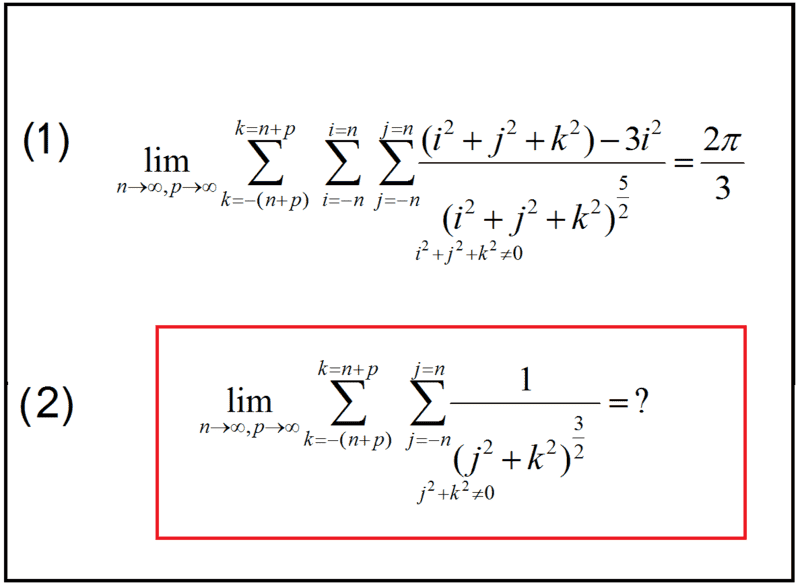# An interesting infinite series

• A
• Hassan2

#### Hassan2While I was was numerically integrating the magnetic field caused by an infinite array of magnetic moments, I observed the interesting limit ( limit (1) in the image). It may seem difficult to prove it mathematically but from the physics point of view, I think it can be proved relatively easily.

If we set i=0 ( no summation on i), the sum seems to have a limit too, but I have not not able find it in a close form ( for example in terms of pi). Any comments?

#### Attachments

Last edited:

(1) is interesting, and I can almost follow what you are doing, (knowing the magnetic field of a magnetic dipole, and it looks like you are computing the x-component of the magnetic field at the origin of something that is magnetized in the x-direction), but what is the geometry of the array? It looks to me like you may be trying to compute the result for a rectangular block, but the geometry that gets the uniform internal field with the well-known result everywhere including the origin is a sphere.

@Charles Link Yes, you got it right! The array is the result of discretizing an infinitely long rectangular prism whose edges are parallel with x, y, and z axis of Cartesian coordinate system. into cubic cells and. The prism is uniformly magnetized in x direction and I have excludes the field due to the central cell itself. Note that the dimension of the prism is infinite in all three dimensions but the prism length is infinitely longer than the other cross-sectional dimensions. This geometry does not give uniform field everywhere and this limit is for the center if the prism. A rectangular prism is somehow similar to an infinitely long cylinder for which the magnetic field is uniform everywhere. The demagnetizing factor of such cylinder is 0.5 and for the prism too, the field in the center is related to the magnetization by factor 0.5.

Last edited:
@Charles Link Yes, you got it right! The array is the result of discretizing an infinitely long rectangular prism whose edges are parallel with x, y, and z axis of Cartesian coordinates system. into cubic cells and. The prism is uniformly magnetized in x direction and I have excludes the field due to the central cell itself. Note that the dimension of the prism is infinite in all three dimensions but the prism length is infinitely longer than the other cross-sectional dimensions. This geometry does not give uniform field everywhere and this limit is for the center if the prism. A rectangular prism is somehow similar to an infinitely long cylinder for which the magnetic field is uniform everywhere. The demagnetizing factor of such cylinder is 0.5 and for the prism too, the field in the center is related to the magnetization by factor 0.5.
Your calculation is rather clever, and essentially you are taking ## \vec{B}(\vec{x})=\int \vec{m}(\vec{x}-\vec{x'}) \, d^3x' ##, where ## \vec{m}(\vec{r}) ## is the field of the magnetic dipole, and you let ## \vec{x}=(0,0,0) ##. ## x=A \frac{i}{n} ##, ## y=A \frac{j}{n} ##, and ## z=A \frac{k}{n} ##, and you selected ## A=1 ##. ## \\ ## A couple of things that I think need correction in your calculation: It is a circular cylindrical prism that has a demagnetizing factor equal to ##\frac{1}{2} ##, and not a square one. Also the sums that you have from what I can tell, make a finite size prism in all directions. You do need to have the x and y be finite, but if you are trying to create the case of the demagnetizing factor of ## \frac{1}{2} ##, you need to somehow make the ## k ## summation so that you indeed have it corresponding to an infinite length in the z-direction, and the limits on the ## i ## and ## j ## sums need to be such as to create a circle in the x-y plane. ## \\ ## Your technique is a clever one, but I don't think you have all the details completely in order yet.

Your technique is a clever one, but I don't think you have all the details completely in order yet.
Nothing very clever here because I just summed up the partial field created by each moment. But since you seem to be well familiar with the physics of the problem, I would like to share with you more details:
I do not really have magnetic moments in the problem. Instead, in a simplified version of the problem, I have a 2D array of straight cylinders immersed in an initially uniform pulsating field. The applied field induces eddy current in the cylinders and the result is an opposing field much like magnetic moments which (not only dipole but also higher poles exists here). I wanted to calculated the effect of neighboring cylinders on the a given cylinder. The limits that I shared here were the result of investigating the convergence of the overall field and I think it is mathematically interesting. Even though the 2D array of cylinders extend to infinity in both cross-sectional directions, the limit still depends on the ratio of dimensions of extensions. For example if we truncate the summation at 2 million neighbors (1 million on each side) in one directions and 4 million neighbors in another direction, the limit would be very different from the case of 2 million neighbors in each direction. This turns out to be related to the demagnetizing factor of the ensemble of cylinders depends on its shape and not the size.

I wish I could find a close form of limit (2) too.